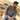# Conditional Statements in JavaScriptTaimoor Sattar

Conditional Statements execute certain operations based on logic. In Javascript, we can execute the conditional statements in three (3) ways as below:

• ternary operator
• if / else logic
• switch statement

let suppose, we have a variable in Javascript as below:

``let isMember = true;``

We can perform condition statements in javascript as below:

ternary operator:

``````// ternary operator
let price= isMember ? '\$2.00' : '\$10.00'``````

if / else logic:

``````// if / else logic
if(isMember){
price = '\$2.00'
}else{
price = '\$10.00'
}``````

switch statement:

``````// switch statement
switch(isMember) {
case true:
price = '\$2.00';
break;
case y:
price = '\$10.00'
break;
default:
price = '\$2.00';
}``````